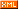# Idiot-maker

:: :: :: :: :: :::: ::291 随笔 :: 0 文章 :: 20 评论 :: 0 引用

https://leetcode.com/problems/bitwise-and-of-numbers-range/

Given a range [m, n] where 0 <= m <= n <= 2147483647, return the bitwise AND of all numbers in this range, inclusive.

Example 1:

Input: [5,7]
Output: 4
Example 2:

Input: [0,1]
Output: 0

The hardest part of this problem is to find the regular pattern.
For example, for number 26 to 30
Their binary form are:
11010
11011
11100
11101
11110

https://leetcode.com/problems/bitwise-and-of-numbers-range/discuss/56729/Bit-operation-solution(JAVA)

class Solution {
public int rangeBitwiseAnd(int m, int n) {
int step = 0;
while (m != n) {
m = m >> 1;
n = n >> 1;
step++;
}
m = m << step;
return m;
}
}

posted on 2018-12-25 22:39 NickyYe 阅读(...) 评论(...) 编辑 收藏